# Custom Image Transformations in fastai

2021-01-02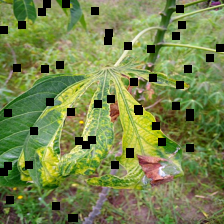# Introduction

Below is the output of an iPython notebook covering the process of transforming images for deep learning applications in the fastai library. In particular, it shows how to use the many transformations in the Albumentations library within a fastai DataBlock.

## A Brief Note

I’ve made a number of these small guides, but haven’t posted them here. I may do so in the future. In general, I want to be better about putting materials I generate here on my site in some format.

## Libraries

Below are the libraries we use throughout this guide. Note that the images themselves come from the Kaggle Cassave Leaf Disease Classification challenge (linked below). That said, with the libraries below, the methods should be applicable to other images. However, the process of loading the preprocessing the images will likely be different.q

import numpy as np # linear algebra
import pandas as pd # data processing, CSV file I/O (e.g. pd.read_csv)
import os
from fastai.vision.all import *
import albumentations as A # the albumentations library has the transformations we will be using


## Minimum Working Code Template

Scroll to the bottom if all you’re interested in is a minimal working code template for creating a transformation that can be passed to a fastai DataBlock.

## Global options

• TEST = True to use only a small subset of images to save time/resources.
TEST = True

def set_seeds():
random.seed(42)
np.random.seed(12345)
torch.manual_seed(1234)
torch.backends.cudnn.deterministic = True
torch.backends.cudnn.benchmark = False


# Data Setup

Nothing new here – the same process as used previously to set up the data for the Cassava competition. We will use only a small subset of the images for testing purposes.

path = Path('../input/cassava-leaf-disease-classification')
train_df['image_id'] = train_df['image_id'].apply(lambda x: f'train_images/{x}')

if TEST: train_df = train_df[0:100] # use only 100 training examples if TEST is True


(                      image_id  label
0  train_images/1000015157.jpg      0
1  train_images/1000201771.jpg      3
2   train_images/100042118.jpg      1
3  train_images/1000723321.jpg      1
4  train_images/1000812911.jpg      3,
(100, 2))


## Making Labels More Interpretable

idx2lbl = {0:"Cassava Bacterial Blight (CBB)",
1:"Cassava Brown Streak Disease (CBSD)",
2:"Cassava Green Mottle (CGM)",
3:"Cassava Mosaic Disease (CMD)",
4:"Healthy"}

train_df['label'].replace(idx2lbl, inplace=True)


image_id label
0 train_images/1000015157.jpg Cassava Bacterial Blight (CBB)
1 train_images/1000201771.jpg Cassava Mosaic Disease (CMD)
2 train_images/100042118.jpg Cassava Brown Streak Disease (CBSD)
3 train_images/1000723321.jpg Cassava Brown Streak Disease (CBSD)
4 train_images/1000812911.jpg Cassava Mosaic Disease (CMD)

# Preparing our Image Transformation(s)

We will be using the albumentations library, which provides many different image transformation options. Our goal, then, is to make the transformations from that library usable within the fastai DataBlock API.

First, we will look at a single example to make sure we can correctly implement the transformation of interest. Here is the base image we’ll be transforming.

img = PILImage.create(path/train_df['image_id'])
img = img.resize((224,224))
img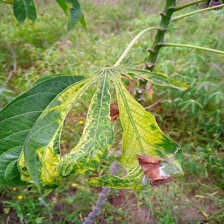## Transformations as Simple Functions

We will begin by defining some simple functions for transforming the images and visualizing the transformations. At this phase, we won’t worry about making them work with the fastai DataBlocks.

We start by defining a generic function that should work for any of the albumentations transforms. This package handles the necessary transformations between data types. We have PILImage images while the package works on numpy images, so we need to convert between types.

def aug_tfm(img):
np_img = np.array(img) # converts image to numpy array
aug_img = aug(image=np_img)['image'] # applies transformation (defined outside of function)
return PILImage.create(aug_img) #returns and visualizes PILImage

aug = A.ToGray(p=1)
aug_tfm(img)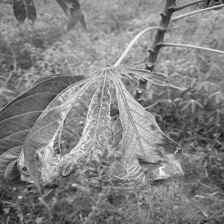### Dropout

aug = A.CoarseDropout(p=1, min_holes = 40, max_holes=50)
aug_tfm(img)### Fog

aug = A.RandomFog(p=1)
aug_tfm(img)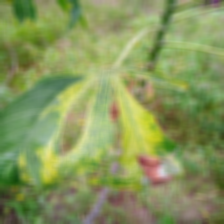## Compositions of Transformations

Multiple transformations can be combined in a single pipeline.

aug = A.Compose([
A.ToGray(p=1),
A.RandomFog(p=1),
A.CoarseDropout(p=1, min_holes = 40, max_holes=50),
])
aug_tfm(img)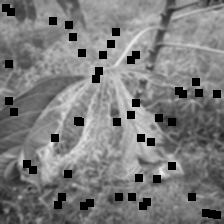# Making these Transformations Work with Fastai

We will now make these transformations work with the fastai DataBlock API. We will demonstrate using the CoarseDropout transformation defined above, as it provides a highly-visible transformation, making it immediately obvious whether the transformation was successfully applied.

## “Baseline” DataBlock

First we show our datablock without any transformations applied.

def get_x(row): return path/row['image_id']
def get_y(row): return row['label']

set_seeds()
db = DataBlock(blocks = (ImageBlock, CategoryBlock),
get_x = get_x,
get_y = get_y,
splitter = RandomSplitter(valid_pct=0.2),
item_tfms = [Resize(224)],
batch_tfms = [*aug_transforms(), Normalize.from_stats(*imagenet_stats)])

bs=10 if TEST else 64
dls.show_batch(max_n = 3, figsize=((12,12)))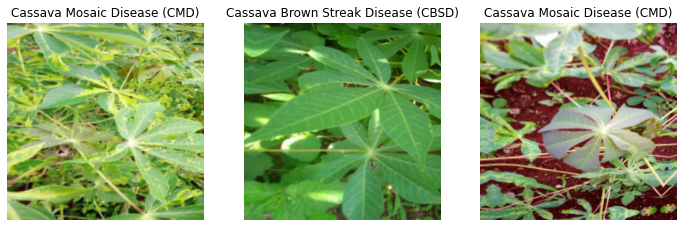## Transformations in the DataBlock

Next, we apply our transformations as item_tfms. To do this, we need to package our transforms into a class that provides a few extra details to the DataBlock.

• split_idx: 0 is for training set; 1 is for validation set; none is for both.
• order tells when to run relative to the other transforms. So order=2 in the example below says to run the transform after the inital resize.

As with the function we defined above, the class we defined below is very modular. We can try out different definitions of aug with the MyTransform class.

aug = A.CoarseDropout(p=1, min_holes = 40, max_holes=50)

class MyTransform(Transform):
split_idx=None #runs on training and valid
order = 2 # runs after initial resize
def __init__(self, aug): self.aug = aug
def encodes(self, img: PILImage):
aug_img = self.aug(image=np.array(img))['image']
return PILImage.create(aug_img)
set_seeds()
db = DataBlock(blocks = (ImageBlock, CategoryBlock),
get_x = get_x,
get_y = get_y,
splitter = RandomSplitter(valid_pct=0.2),
item_tfms = [Resize(224), MyTransform(aug)],
batch_tfms = [*aug_transforms(), Normalize.from_stats(*imagenet_stats)])

bs=10 if TEST else 64
dls.show_batch(max_n = 3, figsize=((12,12)))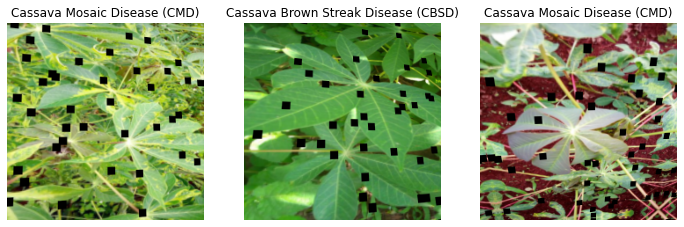Because we specified idx=None, this transformation was applied to the validation set as well.

set_seeds()
dls.valid.show_batch(figsize=((12,12)), max_n = 3)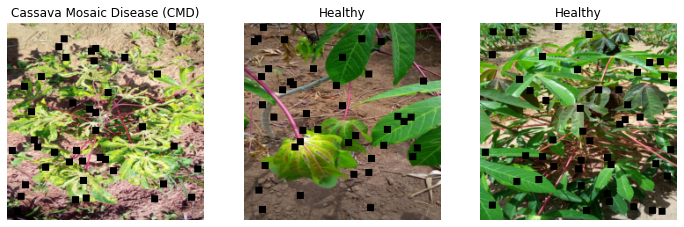Below, we demonstrate that changing the split_idx argument to 0 ensures the transformation is not applied to the validation set.

class MyTransform(Transform):
split_idx=0 #runs on training and valid
order = 2 # runs after initial resize
def __init__(self, aug): self.aug = aug
def encodes(self, img: PILImage):
aug_img = self.aug(image=np.array(img))['image']
return PILImage.create(aug_img)
set_seeds()
db = DataBlock(blocks = (ImageBlock, CategoryBlock),
get_x = get_x,
get_y = get_y,
splitter = RandomSplitter(valid_pct=0.2),
item_tfms = [Resize(224), MyTransform(aug)],
batch_tfms = [*aug_transforms(), Normalize.from_stats(*imagenet_stats)])

bs=10 if TEST else 64
dls.valid.show_batch(max_n = 3, figsize=((12,12)))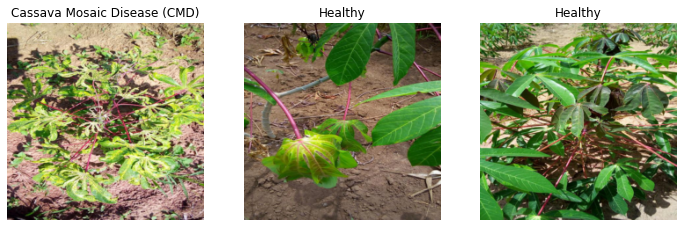## A note note on batch_tfms

I tried to apply this with batch_tfms with no real expectation of it working. The class defined above is clearly defined to work on a single image, not on a batch, so unless there’s some magic happening in the background, I wouldn’t expect it to work.

There is an interesting discussion here on the topic for anyone interested, but for our purposes, sticking with item_tfms is sufficient.

# Minimal Working Code Template

aug = A.CoarseDropout(p=1, min_holes = 40, max_holes=50) # or whatever transform from albumentations you want to use

class MyTransform(Transform):
split_idx=None #runs on training and valid (0 for train, 1 for valid)
order = 2 # runs after initial resize
def __init__(self, aug): self.aug = aug
def encodes(self, img: PILImage):
aug_img = self.aug(image=np.array(img))['image']
return PILImage.create(aug_img)

db = DataBlock(blocks = (ImageBlock, CategoryBlock),
get_x = get_x,
get_y = get_y,
splitter = RandomSplitter(valid_pct=0.2),
item_tfms = [Resize(224), MyTransform(aug)], # put the defined class here.
batch_tfms = [*aug_transforms(), Normalize.from_stats(*imagenet_stats)])